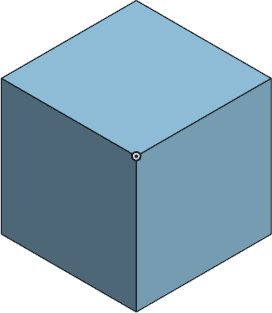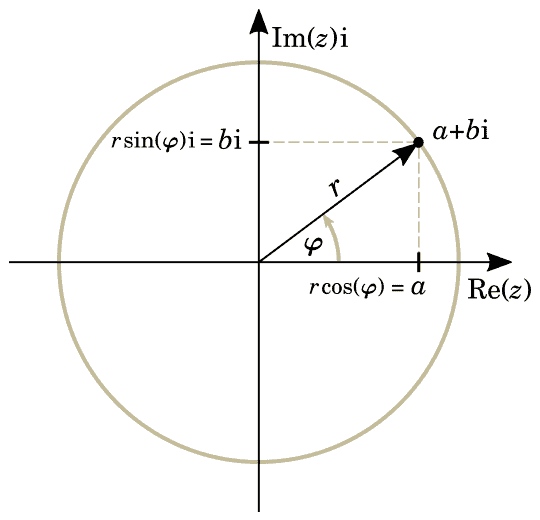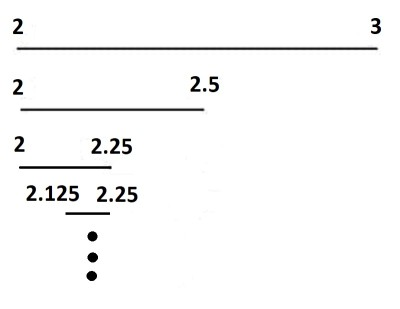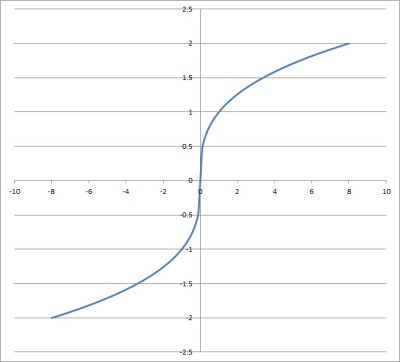# What Is A Cube Root? (7 Common Questions Answered)

A cube root can help us to find the side length of a cube with a given volume.  A cube root can also help us to solve cubic equations (where there is an x3 term).  However, it still helps to know how cube roots work.

So, what is a cube root?  The cube root C of a number N satisfies the equation N = C3 (in words: “N equals C cubed”). The cube root of N is the number C that you multiply by itself 3 times to get N. We can also write C = 3√N to denote the cube root of N.

Of course, when we talk about a cube root of N, we usually mean the principal cube root of N.  Remember that each real number (except zero) has three cube roots: one real cube root and two cube roots that are complex numbers with nonzero imaginary parts.

In this article, we’ll talk about cube roots and answer some common questions about them.  We’ll also look at some examples of how to find cube roots by hand (in case you are ever without a calculator or computer).

Let’s get started.

## What Is A Cube Root?

For a number N, the cube root of N is the number C that satisfies the equation

• N = C3  [N equals C cubed]

We can also write the equation

• C = 3√N  [C equals the cube root of N]

Here is another way to express this idea in words: the cube root of N is the number C that you multiply by itself 3 times to get N.If we know the volume of a cube, we can find a side length by taking the cube root of the volume.

Remember that in addition to the principal cube root of a real number N (which is real), there are two other cube roots (both of which are complex).

For example:

• The principal cube root of -1 is -1 (which is a real number), since (-1)3 = -1.
• Another cube root of -1 is (1/2) + i(√3/2) (which is a complex number).
• Another cube root of -1 is (-1/2) + i(√3/2) (which is a complex number).

Let’s look at a table of the first several real cube roots that are whole number (integers).

### Cube Root Table

The table below shows “perfect cube” integers and their cube roots.

If you memorize the table above, you can recognize perfect cubes when you see them.  This can help you when factoring a sum or difference of cubes.

You can also use this table as a guide when finding cube roots by hand (more on this later).

### Does A Cube Root Have Two Answers?

The cube root of a real number has a single real answer, along with two complex answers.

The real answer is called the principal cube root.We can use complex numbers to write two additional cube roots of a real number N (aside from the principal cube root, which is a real number).

You could solve for all of the complex cube roots of a real number N by solving the equation:

### How To Find A Cube Root By Hand

To find 3√N by hand, start by cubing integers (13 = 1, 23 = 8, 33 = 27, etc.).  Find the first number S such that S3 > N.

Then we know that S – 1 < 3√N < S.  Take the average of S – 1 and S, and then cube it.

If the result is larger than N, try a smaller number; otherwise, try a larger number, and repeat the process.

To recap:

• First, we “bound” 3√N between two whole numbers, S – 1 and S.  We do this by cubing integers (13 = 1, 23 = 8, 33 = 27, etc.) until we find a result S3 that is larger than N.
• Second, we repeatedly “cut in half” the boundary interval for 3√N by taking the average of the endpoints and cubing the result.  If we get something larger than N, we take a smaller number.  If we get something smaller than N, we take a larger number.

It will help to see an example of how this works, so let’s take a look at one.

#### Example: How To Find The Cube Root Of 10 By Hand

Our first step is to bound the cube root of 10 between two whole numbers.

So, we list out perfect cubes until we find one larger than N = 10:

• 13 = 1
• 23 = 8
• 33 = 27

We stop at S = 3, since 33 = 27 is the first cubed integer larger than N = 10.

This means that our boundary is in the interval S – 1 < 3√N < S, or 2 < 3√10 < 3.

In step 2, we find a more exact number for √10 by repeating the following process.

First, take the average of the endpoints of our interval: (2 + 3) / 2 = 2.5.

Next, cube the average we found: 2.53 = 15.625.

Now, since the result of 15.625 is larger than 10, we want a smaller number.

Our boundary is now 2 < 3√10 < 2.5.

Repeating the process:

First, take the average of the endpoints: (2 + 2.5) / 2 = 2.25.

Next, cube the average we found: 2.253 = 729/65, or 11 & (25/64).

Now, since the result of 729/64 is larger than 10, we want a smaller number.

Our boundary is now 2 < 3√10 < 2.25.

Repeating the process:

First, take the average of the endpoints: (2 + 2.25) / 2 = 2.125.

Next, cube the average we found: 2.1253 = 4913/512 or a little less than 10.

Now, since the result of 4913/520 is smaller than 10, we want a larger number.

Our boundary is now 2.125 < 3√10 < 2.25.

Repeating the process:

First, take the average of the endpoints: (2.125 + 2.25) / 2 = 2.1875.

Next, cube the average we found: 2.18753 = 42,875/4,096.

Now, since the result of 42,875/4,096 is larger than 10, we want a smaller number.

Our boundary is now 2.125 < 3√10 < 2.1875.

At this point, the boundary is now only about 1/16 (0.0625) wide.  If we take the average of the two endpoints now, we will get a value that is within 1/32 (0.03125) of 3√10.

If we want more accuracy, we can keep repeating the process above to continue cutting the width of the boundary interval in half.

So, we take (2.125 + 2.1875)/2 = 2 & (5/32) as our value of the cube root of 10.

When cubed, this gives us about 10.025, which is within 0.025 of 10 (an error of about 0.025/10 = 0.25%).We can see the boundary interval get smaller with each step we take to find the cube root of 10 by hand.

(You can learn how to find square roots by hand here).

### Can You Cube Root A Negative Number? (Can A Cube Root Be Negative?)

You can take the cube root of a negative number.  The result will be a negative real number (for the principal cube root).

In other words, a cube root can be negative, but only when we are taking the cube root of a negative number.

If N is a negative real number, then its cube root is –3√|N|.  That is:

• Take the absolute value of N to get |N|, a positive real number.
• Take the principal cube root of |N| to get a positive real number, 3√|N|.
• Take the negative of this positive cube root to get a negative real number, –3√|N|.

For example: to find the cube root of -64:

• Take the absolute value of -64 to get |-64| = 64.
• Take the principal cube root of 64 to get 4.
• Take the negative of this cube root to get -4.

We can check this answer easily:

• (-4)3
• =(-4)*(-4)*(-4)
• =16*(-4)  [product of two negatives is positive]
• =-64  [product of a negative and a positive is negative]

#### Why Is The Cube Root Of A Negative Number Negative?

The cube root of any negative number must be negative, due to the parity (even or odd) of the exponents.

Remember that:

• A negative number raised to an even power is positive.
• A negative number raised to an odd power is negative.
• A positive number raised to any power (even or odd) is positive.

So, the cube root of a negative number must be negative.  A positive number raised to the third power could never give us a negative result.

### Can The Cube Root Of A Positive Number Be Negative?

The cube root of a positive number cannot be negative, due to the parity of the exponents (see above).

A negative number raised to the third power (exponent of 3) must be negative, since 3 is odd.  So, a negative number cubed cannot be positive, and so a positive number cannot have a negative cube root.

### Can You Take The Cube Root Of Zero?

You can take the cube root of zero.  The result is simply zero.  There are two ways to prove this.

First, we can easily show that 03 = 0:

• 03
• =0*0*0
• =0*0
• =0

We can also show that x = 0 is the only solution to the cubic equation x3 = 0:

• x3 = 0
• x*x*x = 0
• x = 0 or x = 0 or x = 0 [x = 0 is a triple real root of the equation]

### Is Cube Root Of X Continuous?

The cube root function f(x) = 3√x is continuous.  We can see its graph below:This is the graph of the cube root function f(x) = 3√x. It is continuous on the real numbers.

However, note that f(x) = 3√x is not differentiable at x = 0, since we get a negative exponent, because:

• f(x) = 3√x
• f(x) = x1/3  [a power of 1/3 is equivalent to taking the cube root]
• f’(x) = (1/3)x-2/3  [by the power rule for taking derivatives]
• f’(x) = 1/(33√(x2))

We can see that x = 0 will give us a zero denominator in the derivative.

## Conclusion

Now you know a little more about cube roots, along with the answers to some common questions about them.  You also know a procedure for finding cube roots by hand, just in case!